Fingerings Home Octave 1: F  (C) Gb  (Db) G  (D) Ab  (Eb) A  (E) Bb  (F) B  (Gb) C  (G) Db  (Ab) D  (A) Eb  (Bb) E  (B) Octave 2+: F  (C) Gb  (Db) G  (D) Ab  (Eb) A  (E) Bb  (F) B  (Gb) C  (G) Db  (Ab) D  (A) Eb  (Bb) E  (B) F  (C) Gb  (Db) G  (D)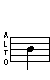B (Gb) - 1st Octave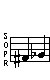Trills

Trill - From Db
(See below for alternate)
Trill - From C
Db B (alt)   C B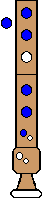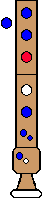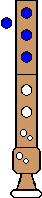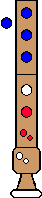Comments: Alternate Db during trill is much too sharp   Comments: Normal fingerings
Alternate Trill - From Db
Db B (alt)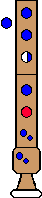Comments: Cover hole 3 less than half. This is very difficult to do. The alternate B will not sound unless hole 3 is partially covered just right.
Top

Turned Trills

Turned Trill
Full step above, Full step below
Db B (alt) A B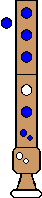Comments: Alternate Db during trill is much too sharp
Top

Turned Trill
Half step above; Full step below
C B A BTop

Turned Trill
Full step above; Half step below
Db B (alt) Bb B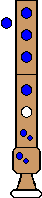Comments: Alternate Db during trill is much too sharp
Top

Turned Trill
Half step above; Half step below
C B Bb (alt) B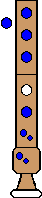Comments: If you're feeling daring, you can use a normal Bb.
Top

Regular Turns

Turn
Full step above; Full step below
Db B A BTop

Turn
Half step above; Full step below
C B A BTop

Turn
Full step above; Half step below
Db B Bb (alt) BComments: If you're feeling brave, use normal Bb
Top

Turn
Half step above; Half step below
C B Bb (alt) BComments: Try using normal Bb too
Top
Note half-step up
Note half-step down
Home